## ORIGINAL RESEARCH article

Front. Built Environ., 22 January 2016
Sec. Earthquake Engineering
https://doi.org/10.3389/fbuil.2016.00001

# Closed-Form Critical Earthquake Response of Elastic–Plastic Structures on Compliant Ground under Near-Fault Ground Motions

• Department of Architecture and Architectural Engineering, Graduate School of Engineering, Kyoto University, Kyoto, Japan

The double impulse is introduced as a substitute of the fling-step near-fault ground motion. A closed-form solution of the elastic–plastic response of a structure on compliant (flexible) ground by the “critical double impulse” is derived for the first time based on the solution for the corresponding structure with fixed base. As in the case of fixed-base model, only the free vibration appears under such double impulse and the energy approach plays an important role in the derivation of the closed-form solution of a complicated elastic–plastic response on compliant ground. It is remarkable that no iteration is needed in the derivation of the critical elastic–plastic response. It is shown via the closed-form expression that in the case of a smaller input level of double impulse to the structural strength, as the ground stiffness becomes larger, the maximum plastic deformation also becomes larger. On the other hand, in the case of a larger input level of double impulse to the structural strength, as the ground stiffness becomes smaller, the maximum plastic deformation becomes larger. The criticality and validity of the proposed theory are investigated through the comparison with the response analysis to the corresponding one-cycle sinusoidal input as a representative of the fling-step near-fault ground motion. The applicability of the proposed theory to actual recorded pulse-type ground motions is also discussed.

## Introduction

As the dense measurement system of earthquake ground motions becomes usual, various aspects of near-fault ground motions have been made clear. Following these situations, the effects of near-fault ground motions on structural response have been studied extensively (Bertero et al., 1978; Hall et al., 1995; Sasani and Bertero, 2000; Alavi and Krawinkler, 2004; Makris and Black, 2004; Mavroeidis et al., 2004; Kalkan and Kunnath, 2006, 2007; Xu et al., 2007; Rupakhety and Sigbjörnsson, 2011; Yamamoto et al., 2011; Minami and Hayashi, 2013; Khaloo et al., 2015; Vafaei and Eskandari, 2015). The versatile investigations clarified the fling-step and forward-directivity characteristics (Mavroeidis and Papageorgiou, 2003; Bray and Rodriguez-Marek, 2004; Kalkan and Kunnath, 2006, 2007; Mukhopadhyay and Gupta, 2013a,b; Zhai et al., 2013; Hayden et al., 2014; Yang and Zhou, 2014). In particular, Northridge earthquake in 1994, Hyogoken-Nanbu (Kobe) earthquake in 1995, and Chi-Chi (Taiwan) earthquake in 1999 induced strong attention to many earthquake structural engineers and designers.

Some researchers modeled the fling-step and forward-­directivity inputs by a few wavelets or a series of harmonic waves. In this modeling, many useful research works have been conducted. Mavroeidis and Papageorgiou (2003) studied the characteristics of this class of ground motions in detail and constructed some simple models (for example, Gabor wavelet and Berlage wavelet). Xu et al. (2007) used a kind of Berlage wavelet for the performance evaluation of passive energy dissipation systems. Takewaki and Tsujimoto (2011) employed the Xu’s approach and proposed a method for scaling ground motions by taking the drift and input energy demand into account. Takewaki et al. (2012) used a sinusoidal wave for pulse-type waves.

While the closed-form or nearly closed-form solutions of the elastic–plastic earthquake response have been obtained so far only for the steady-state response to sinusoidal input or the transient response to an extremely simple sinusoidal input (Caughey, 1960a,b; Roberts and Spanos, 1990; Liu, 2000), Kojima and Takewaki (2015a,b) demonstrated that the elastic–plastic response (continuation of free vibrations) can be derived by an energy approach without solving directly the equations of motion as differential equations.

The resonance plays a key role in the earthquake-resistant design and it has a strong effect even in case of near-fault ground motions. In the previous research, the resonant equivalent frequency had to be computed for a specified input level by changing the excitation frequency in a parametric manner (Caughey, 1960a,b; Roberts and Spanos, 1990; Liu, 2000). On the contrary, no iteration is required in the recently proposed method for the double impulse (Kojima and Takewaki, 2015a). They demonstrated that the resonance can be proved by using energy investigation, and the critical timing of the second impulse can be characterized as the time with zero restoring force. This advantageous feature is retained also in this paper for the structures on flexible ground. They also made clear that the maximum elastic–plastic response after impulse can be obtained by equating the initial kinetic energy computed by the initial velocity to the sum of hysteretic and elastic strain energies. It should be reminded that while most of the previous researches on near-fault ground motions are aimed at disclosing the response characteristics of elastic or elastic–plastic structures with arbitrary stiffness and strength parameters and require tremendous amount of numerical task, the present paper focused on the critical response (resonant response) and enabled the drastic reduction of computational works.

The double impulse is introduced as a substitute of the representative near-fault ground motion and a closed-form solution of the elastic–plastic response of a structure on compliant ground by the “critical double impulse” is derived based on the solution for the corresponding structure with fixed base. As in the case of fixed-base model, an energy approach is shown to play an important role in the derivation of the closed-form solution of a complicated elastic–plastic response on compliant ground. It is shown that in the case of a smaller input level, as the ground stiffness becomes larger, the maximum plastic deformation also becomes larger. On the other hand, in the case of a larger input level, as the ground stiffness becomes smaller, the maximum plastic deformation becomes larger. These properties are explained by the strain energy stored in the swaying and rocking springs representing the ground stiffness. The energy balance law leads to a larger plastic deformation in the stiff ground with smaller strain energy at a smaller input level. On the other hand, the soft ground with larger strain energy just before the second impulse provides a larger plastic deformation at a larger input level. The criticality and validity of the proposed theory are investigated through the comparison with the response analysis to the corresponding one-cycle sinusoidal input as a representative of the fling-step near-fault ground motion.

The applicability of the proposed method using the double impulse to actual recorded pulse-type ground motions is also investigated.

## Double Impulse Input

### Double Impulse Input

As explained in the previous papers (Kojima and Takewaki, 2015a,b; Kojima et al., 2015), the fling-step input (fault-parallel) of the near-fault ground motion can be represented by a one-cycle sinusoidal wave and the forward-directivity input (fault-normal) of the near-fault ground motion can be expressed by a series of three sinusoidal wavelets (see Figure 1). The fling step is caused by the permanent displacement of the ground induced by the fault dislocation and the forward-directivity effect is concerned with the relation of the movement of the rupture front with the site. In this paper, it is intended to simplify typical near-fault ground motions by a double impulse (Kojima and Takewaki, 2015a; Kojima et al., 2015). This is because the double impulse has a simple characteristic and a straightforward expression of the response can be expected even for elastic–plastic responses based on an energy approach to free vibrations. Furthermore, the double impulse enables us to describe directly the critical timing of impulses (resonant frequency), which is not easy for the sinusoidal and other inputs without a repetitive procedure. It is remarkable to note that, while most of the previous methods employ the equivalent linearization of the structural model with the input unchanged (see Figure 2A including an equivalent linear stiffness), the method proposed in the works (Kojima and Takewaki, 2015a,b) and in this paper transforms the input into the double impulse with the structural model unchanged (see Figure 2B).

FIGURE 1
FIGURE 2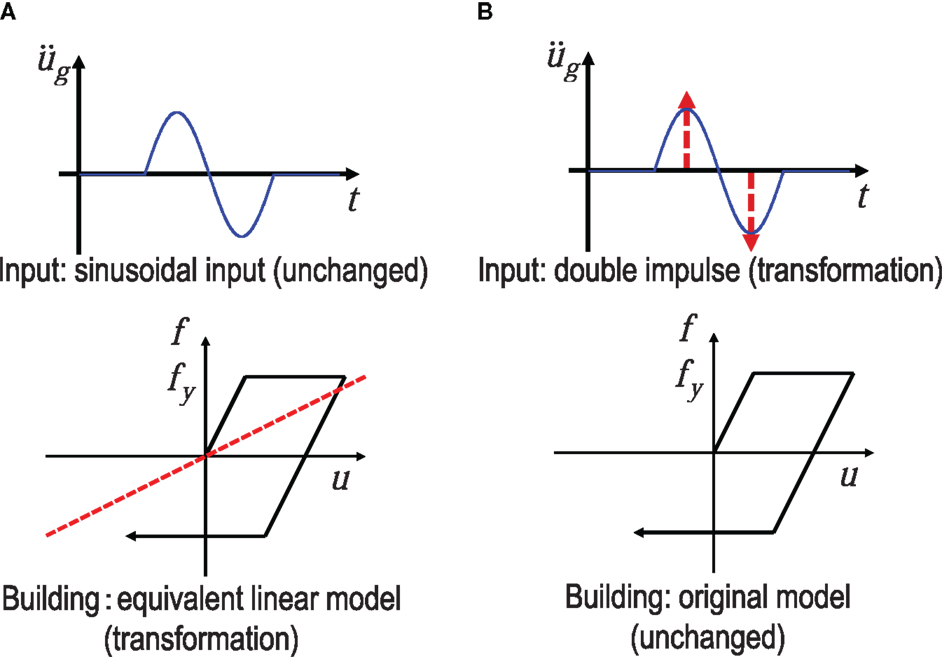Figure 2. Comparison of the proposed method with the previous method: (A) previous method (equivalent linearization of the structural model with the input unchanged) and (B) proposed method (transformation of the input into the double impulse with the structural model unchanged).

Consider a ground acceleration $u¨g(t)$ as double impulse, as shown in Figure 1A, expressed by

$u¨g(t)=Vδ(t)−Vδ(t−t0)$

where V is the given initial velocity and t0 is the time interval between two impulses. The comparison with the corresponding one-cycle sinusoidal wave as a representative of the fling-step input of the near-fault ground motion (Mavroeidis and Papageorgiou, 2003; Kalkan and Kunnath, 2006) is plotted in Figure 1A. The corresponding velocity and displacement of such double impulse and sinusoidal wave are also plotted in Figure 1A. Those for the triple impulse are shown in Figure 1B for reference. It can be understood that the double impulse is a good approximation of the corresponding sinusoidal wave even in the form of velocity and displacement. However, the correspondence in the response should be discussed carefully. This will be conducted in Section “Better Correspondence between Double Impulse and Sinusoidal Input.”

The Fourier transform of $u¨g(t)$ of the double impulse can be derived as

$U¨g(ω)=∫ −∞ ∞{Vδ(t)−Vδ(t−t0)}e−iωtdt =V(1−e−iωt0)$

### Previous Work on Closed-Form Critical Elastic–Plastic Response of SDOF System Subjected to Double Impulse

In the previous work (Kojima and Takewaki, 2015a), a closed-form expression of the critical elastic–plastic response of an SDOF system has been derived for the double impulse. The critical response plays a key role in the worst-case analysis (Drenick, 1970; Takewaki, 2002, 2007; Moustafa et al., 2010; Takewaki et al., 2012). Since this expression is used effectively in this paper, the essence will be shown in this section.

Consider an undamped elastic-perfectly plastic SDOF system of mass m and stiffness k. The yield deformation and yield force are denoted by dy and fy (see Figure 3). Let $ω1=k/m$, u, and f denote the undamped natural circular frequency, the displacement of the mass relative to the ground, and the restoring force of the model, respectively. The plastic deformation after the first impulse is expressed by up1 and that after the second impulse is denoted by up2. The time derivative is denoted by an overdot.

FIGURE 3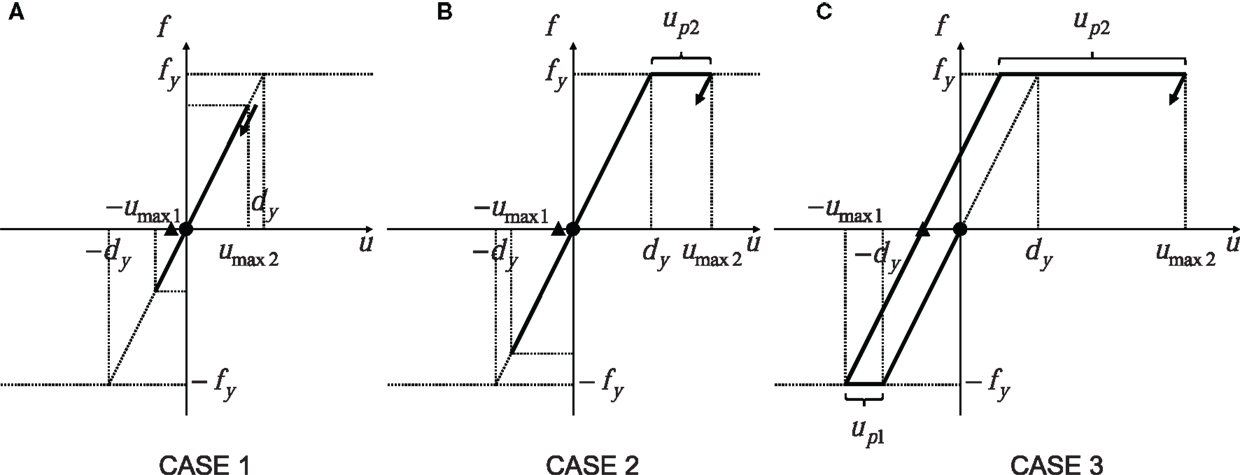Figure 3. Prediction of maximum elastic–plastic deformation under double impulse based on energy approach: (A) Case 1: elastic response; (B) Case 2: plastic response after the second impulse; and (C) Case 3: plastic response after the first impulse (•: first impulse, ▲: second impulse).

The impulse input changes the mass velocity by V instantaneously and the elastic–plastic response of the SDOF system under the double impulse can be expressed by the continuation of free vibrations. Let umax1 and umax2 denote the maximum deformation after the first impulse and that after the second impulse, respectively, as shown in Figure 3. Those responses can be derived by an energy approach without solving directly the differential equation (equation of motion). The kinetic energy given at the initial stage (the time of the first impulse) and at the time of the second impulse is transformed into the sum of the hysteretic energy and the strain energy corresponding to the yield deformation. It should be noted that the critical timing of the second impulse corresponds to the state with a zero restoring force and only a kinetic energy exists in this state as mechanical energies. By using this rule, the maximum deformation can be obtained in a simple manner.

The maximum elastic–plastic response of the SDOF system under the critical double impulse can be classified into the three cases depending on the yielding stage. Let Vy (=ω1dy) denote the input level of velocity of the double impulse at which the SDOF system just attains the yield deformation after the first impulse. This parameter also presents a strength parameter of the SDOF system. Case 1 indicates the case of elastic response even after the second impulse and Case 2 implies the case of plastic deformation only after the second impulse. In addition, Case 3 presents the case of plastic deformation after the first impulse. Figure 3 shows the schematic diagram for these three cases.

Figure 3A shows the maximum deformation after the first impulse and that after the second impulse, respectively, for the elastic case (CASE 1) during the whole stage. umax1 and umax2 can be obtained as follows from the energy balance.

$umax1/dy=V/Vy$
$umax2/dy=2(V/Vy)$

Following the similar energy balance law, umax1 and umax2 for the Cases 2 and 3 (Figures 3B,C) can be obtained as follows:

$umax1/dy=V/Vy(Case 2)$
$umax2/dy=0.5{1+(2V/Vy)2}(Case 2)$
$umax1/dy=0.5{1+(V/Vy)2}(Case 3)$
$umax2/dy=0.5(3+2V/Vy)(Case 3)$

Figure 4 shows the maximum normalized elastic–plastic deformation under the double impulse with respect to input level.

FIGURE 4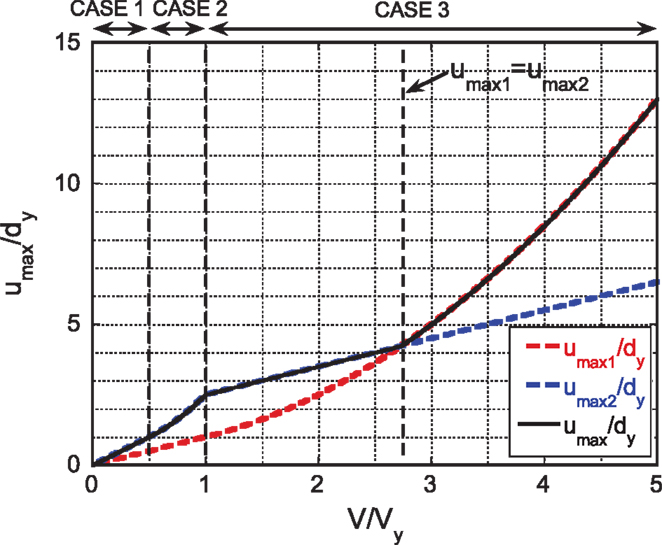Figure 4. Maximum normalized elastic–plastic deformation under double impulse with respect to input level (Kojima and Takewaki, 2015a).

## Maximum Elastic–Plastic Deformation of Simplified Swaying-Rocking Model Subjected to Critical Double Impulse

### Simplified Swaying-Rocking Model

In this paper, a closed-form expression (Kojima and Takewaki, 2015a) of the maximum elastic–plastic response of an SDOF system under the critical double impulse is used in order to derive the closed-form expression of the maximum elastic–plastic response of a simplified swaying-rocking (SR) model, as shown in Figure 5A, under the critical double impulse. In the simplified SR model, the foundation mass and floor mass moments of inertia are neglected in the original SR model.

FIGURE 5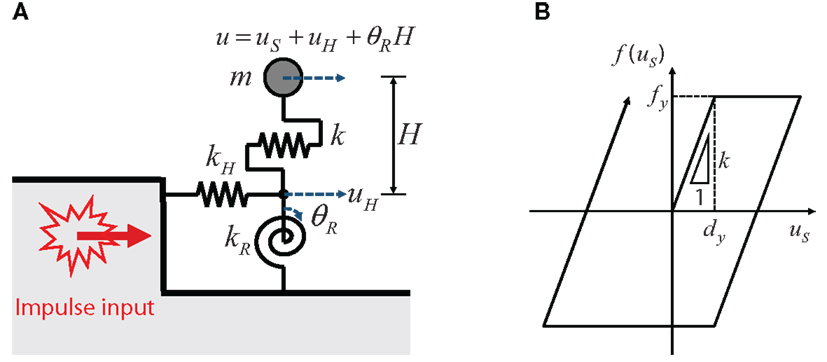Figure 5. Simplified swaying-rocking model: (A) model parameters and (B) restoring force characteristic.

The superstructure is the same as explained in Section “Previous Work on Closed-Form Critical Elastic–Plastic Response of SDOF System Subjected to Double Impulse.” Let kH and kR denote the swaying spring stiffness and the rocking spring stiffness, respectively. The restoring force characteristic is shown in Figure 5B. The damping of the superstructure and the ground is neglected here for simple explanation of the effect of the ground stiffness on the maximum elastic–plastic response of the superstructure. Let uS, uH, and θR denote the actual deformation of the superstructure, the swaying spring deformation and the angle of rotation of the rocking spring. H denotes the equivalent height of the superstructure mass.

In this paper, the swaying and rocking spring stiffnesses are assumed to be expressed by

$kH={6.77/(1.97−ν)}Gr$
$kR={2.52/(1.00−ν)}Gr3$

where ν, r, G, ρ, and VS denote the Poisson’s ratio of the ground, the equivalent radius of the foundation, the shear modulus of the ground, the mass density of the ground and the shear wave velocity of the ground (Parmelee, 1970).

The equations of motion for the simplified SR model in the elastic range can be expressed by

$m(u¨S+u¨H+θ¨RH)+kuS=−mu¨g$
$kuS−kHuH=0$
$kuSH−kRθR=0$

On the other hand, the equations of motion for the simplified SR model in the elastic–plastic range can be described by

$m(u¨S+u¨H+θ¨RH)+f(uS)=−mu¨g$
$f(uS)−kHuH=0$
${f(uS)}H−kRθR=0$

where f(uS) is the restoring force in the superstructure.

### Equivalent SDOF Model of Simplified Swaying-Rocking Model

In order to use the expression in Section “Previous Work on Closed-Form Critical Elastic–Plastic Response of SDOF System Subjected to Double Impulse,” consider an equivalent SDOF model of the simplified SR model as shown in Figure 6A. The equation of motion of the equivalent SDOF model in the elastic range can be expressed by

$mu¨e+keue=−mu¨g$

where ue and ke are the displacement of the mass of the equivalent SDOF model and the elastic stiffness of the equivalent SDOF model. Since the three springs are connected in series, ke can be expressed as follows in terms of the stiffnesses of the three springs (Veletsos and Meek, 1974; Veletsos, 1977; Jarernprasert et al., 2013).

FIGURE 6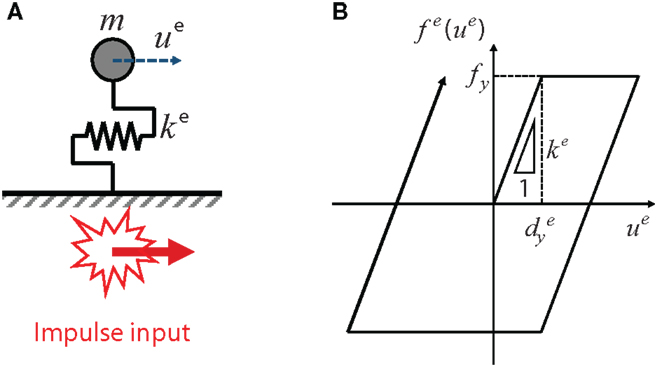Figure 6. Equivalent SDOF model of simplified swaying-rocking model: (A) model parameters and (B) restoring force characteristic.

$ke=k/{1+(k/kH)+(k/kR)H2}$

Because the yield force in the equivalent SDOF model is equal to the yield force of the superstructure, the yield displacement of the equivalent SDOF model can be described as

$dy e=fy/ke={1+(k/kH)+(k/kR)H2}dy$

In addition, the natural frequency of the equivalent SDOF model is computed as

$ω1 e=ke/m=1/{1+(k/kH)+(k/kR)H2}ω1$

Using Eqs 15 and 16, the reference input level corresponding to Vy (=ω1dy) for the superstructure can be defined as

$Vy e=ω1 edy e={1+(k/kH)+(k/kR)H2}ω1dy={1+(k/kH)+(k/kR)H2}Vy$

After the simplified SR model is transformed into the equivalent SDOF model that is defined in Figure 6, the maximum elastic–plastic response of the equivalent SDOF model under the critical double impulse can be obtained as shown in Figure 7 by replacing the parameters with the equivalent parameters.

FIGURE 7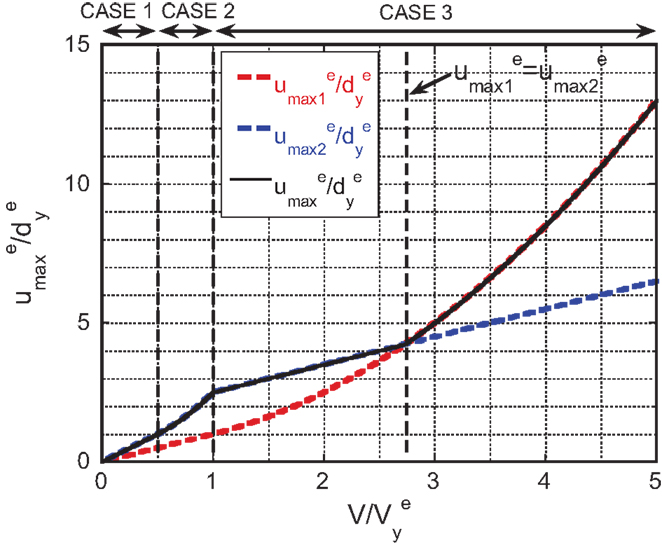Figure 7. Maximum deformation of equivalent SDOF model with respect to the input level normalized for equivalent SDOF model.

### Critical Elastic–Plastic Response of Simplified Swaying-Rocking Model Subjected to Double Impulse

In this section, the maximum elastic–plastic response of the superstructure in the simplified SR model under the critical double impulse is derived. The ductility factor of the superstructure can be expressed by (dy + up)/dy. Although the ductility factor of the superstructure can be obtained from the ductility factor of the equivalent SDOF model introduced in Section “Equivalent SDOF Model of Simplified Swaying-Rocking Model” by modifying the coefficient, the direct expression of the ductility factor of the superstructure is derived here.

As in Section “Previous Work on Closed-Form Critical Elastic–Plastic Response of SDOF System Subjected to Double Impulse,” the three cases are treated depending on the input level of the double impulse, i.e., Case 1, Case 2, and Case 3.

First of all, consider Case 1 (see Figure 8A). The energy balances after the first impulse and the second impulse are expressed as follows:

$mV2/2=kuSmax1 2/2+kHuH1 2/2+kRθR1 2/2$
$m(2V)2/2=kuSmax2 2/2+kHuH2 2/2+kRθR2 2/2$

where uSmax1, uH1, and θR1 denote the maximum deformations of the superstructure, the swaying spring, and the rocking spring, respectively, after the first impulse, and uSmax2, uH2, and θR2 denote those after the second impulse. uH1, θR1, uH2, and θR2 in the elastic range can be expressed as follows in terms of uSmax1 and uSmax2.

$uH1=(k/kH)uSmax1, θR1=(kH/kR)uSmax1$
$uH2=(k/kH)uSmax2, θR2=(kH/kR)uSmax2$
FIGURE 8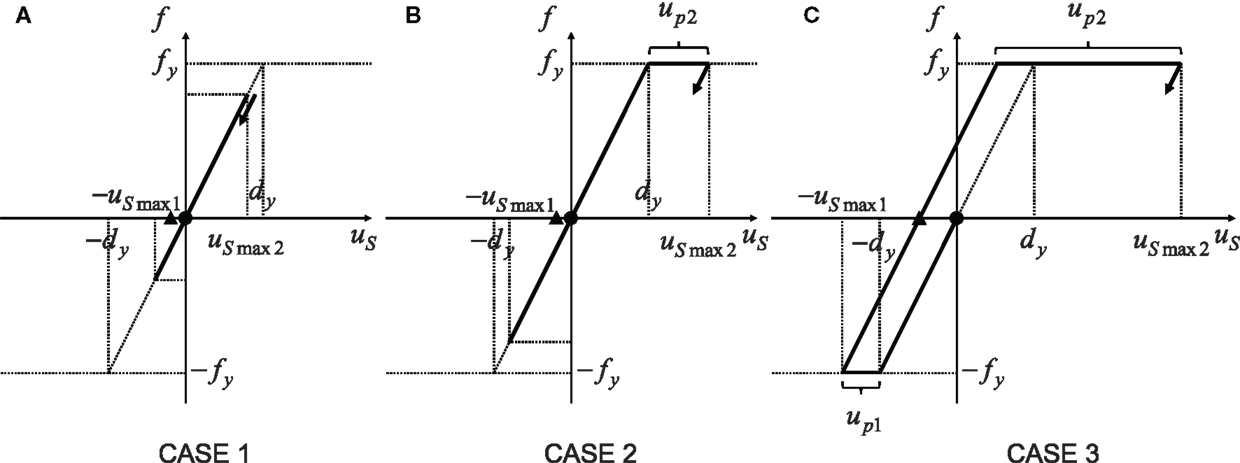Figure 8. Prediction of maximum elastic–plastic deformation of superstructure on compliant ground under double impulse based on energy approach: (A) Case 1: elastic response; (B) Case 2: plastic response after the second impulse; and (C) Case 3: plastic response after the first impulse (•: first impulse, ▲: second impulse).

Substitution of Eqs 20a,b and 21a,b into Eqs 18 and 19 and rearrangement of the resulting equations provide

$uSmax1=1/{1+(k/kH)+(kH2/kR)}(V/ω1)$
$uSmax2=1/{1+(k/kH)+(kH2/kR)}(2V/ω1)$

Dividing both sides of Eqs 22 and 23 by dy and taking into account the relation Vy = ω1dy, the following expressions are derived.

$uSmax1/dy=1/{1+(k/kH)+(kH2/kR)}(V/Vy)$
$uSmax2/dy=1/{1+(k/kH)+(kH2/kR)}(2V/Vy)$

where Vy is the reference input level for the fixed-base superstructure introduced in Section “Previous Work on Closed-Form Critical Elastic–Plastic Response of SDOF System Subjected to Double Impulse” and indicates the strength of the superstructure.

Figure 8B shows the elastic–plastic deformation of the superstructure after the second impulse in Case 2 (the superstructure goes into the plastic range after the second impulse). From Eq. 25, the condition that the superstructure goes into the plastic range after the second impulse can be expressed as

$V/Vy>0.51+(k/kH)+(kH2/kR)$

In Case 2, the superstructure is in the elastic range between the first impulse and the second impulse. The energy balance after the second impulse can be expressed as follows by using the energy balance law and Figure 8B.

$m(2V)2/2=fydy/2+fyup2+kHuH2 2/2+kRθR2 2/2$

where up2, uH2, and θR2 are the maximum plastic deformation of the superstructure and the maximum deformations of the swaying and rocking springs. The restoring force of the superstructure in the plastic range after the second impulse is expressed by

$f(uS)=fy$

uH2 and θR2 can be derived from Eqs 12b,c and 28.

$uH2=fy/kH=(k/kH)dy, θR2=(fyH)/kR=(kH/kR)dy$

Then, up2 can be obtained by substituting Eqs 29a,b into Eq. 27 and rearranging the resulting equation.

$up2=0.5[{(2V)/(ω1dy)}2−{1+(k/kH)+(kH2/kR)}]dy$

Dividing both sides of Eq. 30 by dy and recalling ω1dy = Vy, the following relation is derived.

$up2/dy=0.5[{(2V)/Vy}2−{1+(k/kH)+(kH2/kR)}]$

Figure 8C shows the plastic deformations after the first and second impulses in Case 3 (the superstructure goes into the plastic range after the first impulse). The condition that the superstructure goes into the plastic range after the first impulse can be derived from Eq. 24.

$V/Vy>1+(k/kH)+(kH2/kR)$

Using the energy balance law and Figure 8C, the energy balances after the first and second impulses can be expressed as

$mV2/2=fydy/2+fyup1+kHuH1 2/2+kRθR1 2/2$
$m(vc+V)2/2=fydy/2+fyup2+kHuH2 2/2+kRθR2 2/2$

where up1, uH1, and θR1 denote the maximum plastic deformation of the superstructure and the maximum deformations of the swaying and rocking springs after the first impulse and up2, uH2, and θR2 are those after the second impulse. The restoring force in the superstructure can be expressed as

$f(uS)=−fy$

uH1 and θR1 can be obtained from Eqs 12b,c and 35.

$uH1=−fy/kH=−(k/kH)dy, θR1=−(fyH)/kR=−(kH/kR)dy$

uH2 and θR2 in Case 3 are the same as in Eq. 29. vc in Eq. 34 is the superstructure mass velocity when the restoring force of the superstructure becomes 0 after the first impulse. The energy balance after the initiation of unloading before the second impulse provides

$fydy/2+kHuH1 2/2+kRθR1 2/2=mvc 2/2$

Substitution of Eq. 36 into Eq. 37 and rearrangement of the resulting equation lead to the following expression of vc.

$vc=1+(k/kH)+(kH2/kR)(ω1dy)=1+(k/kH)+(kH2/kR)Vy$

Substituting Eqs 29 and 36 into Eqs 33 and 34 and rearranging the resulting equations, the following expressions can be drawn.

$up1=0.5[{V/(ω1dy)}2−{1+(kH2/kR)+(k/kH)}]dy$
$up2=0.5[{(vc+V)/(ω1dy)}2−{1+(kH2/kR)+(k/kH)}]dy$

Dividing both sides of Eqs 39 and 40 and using Eq. 38 and the relation ω1dy = Vy, the following relations can be obtained.

$up1/dy=0.5[(V/Vy)2−{1+(kH2/kR)+(k/kH)}]$
$up2/dy=0.5(V/Vy)2+1+(kH2/kR)+(k/kH)(V/Vy)$

After the comparison of Eqs 41 and 42, up2/dy > up1/dy can be confirmed. Therefore, we use up/dy = up2/dy. Finally, up/dy can be derived as follows:

$up/dy={0.5{(2VVy)2−(1+kkH+kH2kR)} for 0.51+kkH+kH2kR≤V/Vy<1+kkH+kH2kR0.5(VVy)2+1+kkH+kH2kR(VVy) for 1+kkH+kH2kR≤V/Vy$

### Numerical Example

In this section, the effect of soil types on the response of superstructures is investigated using the closed-form expressions in the previous section.

Consider three soil conditions, soil type 1, 2, and 3. The shear wave velocities of soil type 1, 2, and 3 are VS = 200 m/s for soil type 1, VS = 133 m/s for soil type 2, and VS = 100 m/s for soil type 3. The mass of the superstructure is m = 800 × 103 kg and the natural period of the superstructure with fixed base is 1.0 s. The superstructure is modeled from a 10-story building. The yield deformation is dy = 0.16 m, the equivalent height is H = 40 × 0.7 = 28 m, and the equivalent radius of the foundation is r = 8 m. The mass density of ground is ρ = 1.8 × 103 kg/m3 and the Poisson’s ratio is ν = 0.35.

Figure 9 shows the relation of (dy + up)/dy with V/Vy for three soil conditions and fixed-base case. V is the initial velocity. In the present numerical example, (dy + up)/dy derived from Eq. 43 is treated and the input level is normalized for Vy = ω1dy of the superstructure with fixed base.

FIGURE 9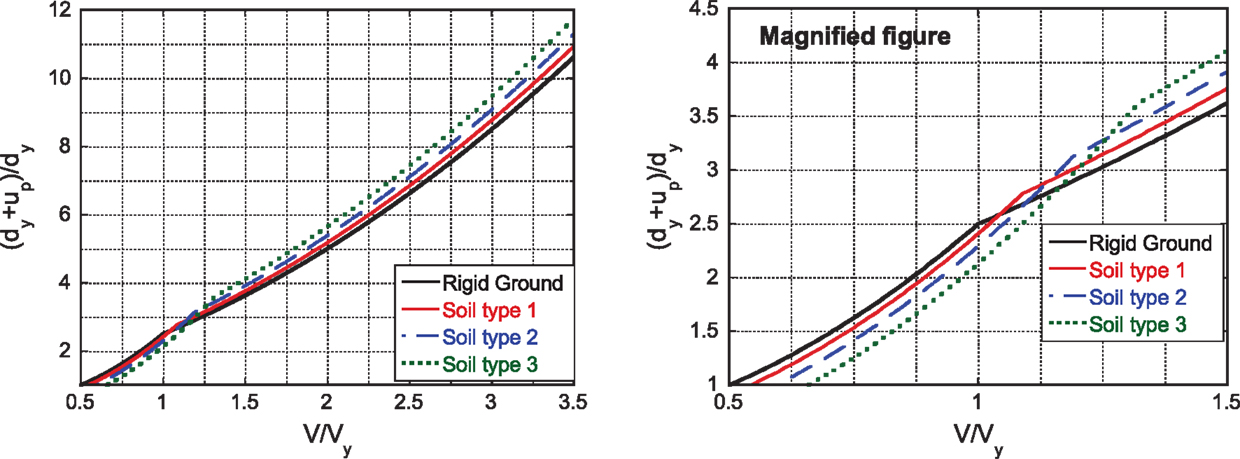Figure 9. Relation of the maximum plastic deformation (dy + up)/dy with V/Vy for three soil conditions and fixed-base case.

The following observations can be drawn. In Case 2 (low input level), as the ground becomes stiffer, the plastic deformation of the superstructure becomes larger. On the other hand, in Case 3 (large input level), as the ground becomes softer, the plastic deformation of the superstructure becomes larger. These properties result from the fact that, as the ground becomes softer, the strain energy stored in the ground becomes larger in the case where the superstructure is in the plastic range.

In more detail, in Case 2, the input energy (the left-hand side of Eq. 27) at the second impulse is constant. As the ground becomes stiffer, the strain energy (the third and fourth terms of the right-hand side of Eq. 27) stored in the ground becomes smaller and the plastic deformation (the second term of the right-hand side of Eq. 27) becomes larger (see Figure 10A).

FIGURE 10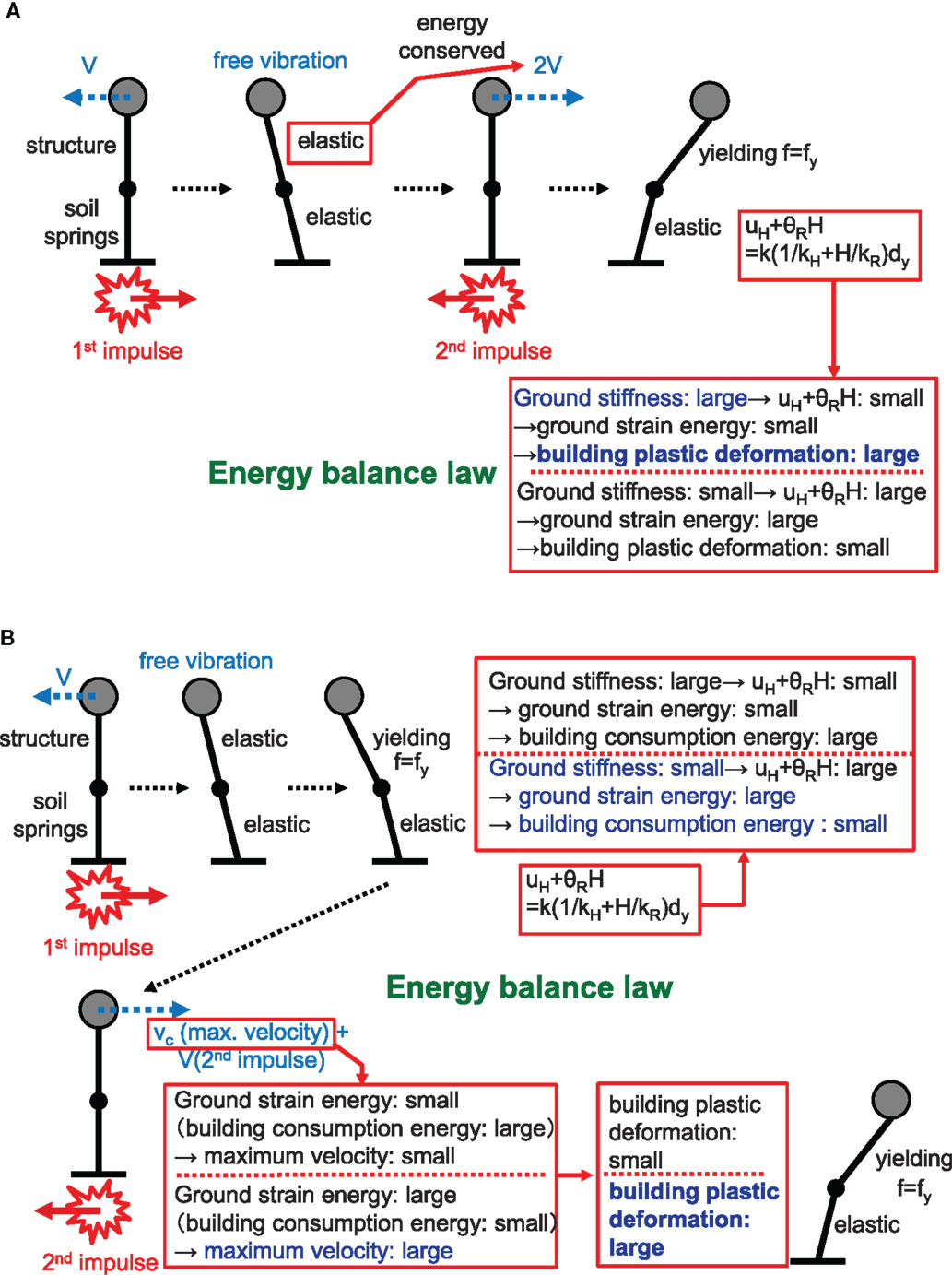Figure 10. Schematic diagram for better understanding of effect of soil type on superstructure plastic deformation: (A) small input level (Case 2) and (B) large input level (Case 3).

On the other hand, in Case 3, the mass velocity (the right-hand side of Eqs 37 and 38) just before the second impulse becomes larger from Eq. 37 as the ground becomes softer (as the strain energy (the second and third terms of the left-hand side of Eq. 37) stored in the ground becomes larger). Then, the input energy (the left-hand side of Eq. 34) at the second impulse becomes larger and the plastic deformation after the second impulse becomes larger (see Figure 10B). This phenomenon results from the fact that the velocity is included in the form of second order in Eq. 34. This result can also be understood from the difference of the second terms in Eq. 43. It can be said that the closed-form expression in Eq. 43 is very useful in the clarification of the effect of soil types on the superstructure response. It should be remarked that the present formulation disregarded the damping of ground. Since the damping is larger in the soft ground, the values in Figure 9 become smaller for softer ground in general. However, it is also true that the ground damping is less effective under impulsive inputs. These influences will be studied in the future.

## Applicability of Critical Double Impulse Timing to Corresponding Sinusoidal Wave

In the previous paper (Kojima and Takewaki, 2015a), it has been demonstrated that if the maximum value of the Fourier amplitude is selected as the key parameter, the responses to the double impulse and the corresponding sinusoidal input exhibit a fairly good correspondence. In this section, it is investigated whether the critical timing derived from the double impulse is also an approximate critical timing of the sinusoidal input. Although the SDOF model with fixed base is treated here, it is applicable to the equivalent SDOF model by introducing the equivalent parameters, $Vy e,dy e,ω1 e$, etc.

Let $t0c$ denote the critical timing of the double impulse and t0 denote the general timing. In the previous paper (Kojima and Takewaki, 2015a), $t0c$ has been derived as follows:

$t0c/T1={arcsin(Vy/V)}/(2π)+{(V/Vy)2−1/(2π)}+1/4$

This relation is plotted in Figure 11. It can be observed that the critical timing is delayed due to plastic deformation as the input level increases.

FIGURE 11

Figure 12A shows the maximum deformation with respect to $t0/t0c$. It can be observed that $t0c$ derived from the double impulse is a good approximate of the critical timing for the sinusoidal input. Figure 12B is the corresponding plot for the double impulse (Kojima and Takewaki, 2015a).

FIGURE 12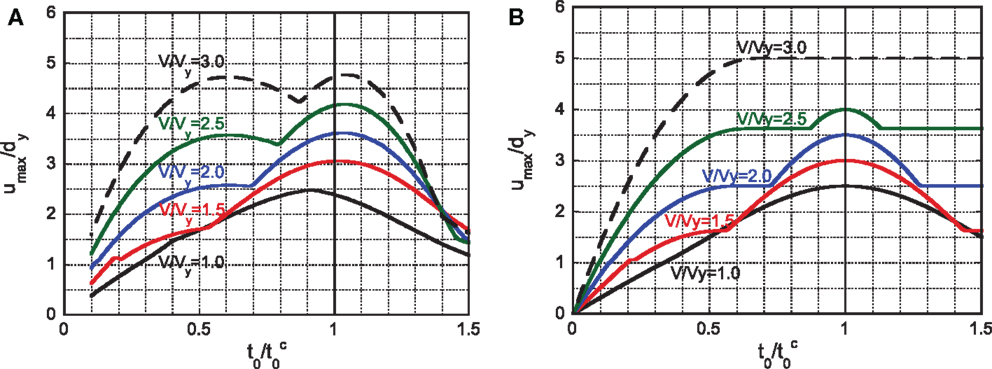Figure 12. Maximum deformation with respect to $t0/t0c$: (A) sinusoidal input and (B) double impulse.

## Better Correspondence Between Double Impulse and Sinusoidal Input

In the previous work (Kojima and Takewaki, 2015a), it has been made clear that if the magnitude of the double impulse is adjusted so that the maximum values of the Fourier amplitudes of the double impulse and the corresponding sinusoidal input are the same, the maximum elastic–plastic responses correspond well in the range of input level V/Vy < 3. However, in the range of V/Vy > 3, the maximum response of the double impulse becomes larger than that of the sinusoidal input. In order to investigate the better correspondence over a wider range, the amplitude of the sinusoidal input is amplified. As in Section “Applicability of Critical Double Impulse Timing to Corresponding Sinusoidal Wave,” although the SDOF model with fixed base is treated here, it is applicable to the equivalent SDOF model by introducing the equivalent parameters, $Vy e,dy e,ω1 e$, etc.

Figure 13A shows the plot of the coefficient a with respect to the timing of the double impulse for adjusting the maximum Fourier amplitudes of the double impulse and the sinusoidal input where the sinusoidal acceleration input is expressed as $u¨g(t)=Asin(πt/t0)$ and the coefficient a is defined by a = A/V. Figures 13B–E present the maximum normalized elastic–plastic deformations to the double impulse and the corresponding sinusoidal inputs amplified by 1.0, 1.1, 1.15, and 1.2 from the original input with the same maximum Fourier amplitude as the double impulse. It can be observed that the amplification 1.15 or 1.2 provide the best fitting.

FIGURE 13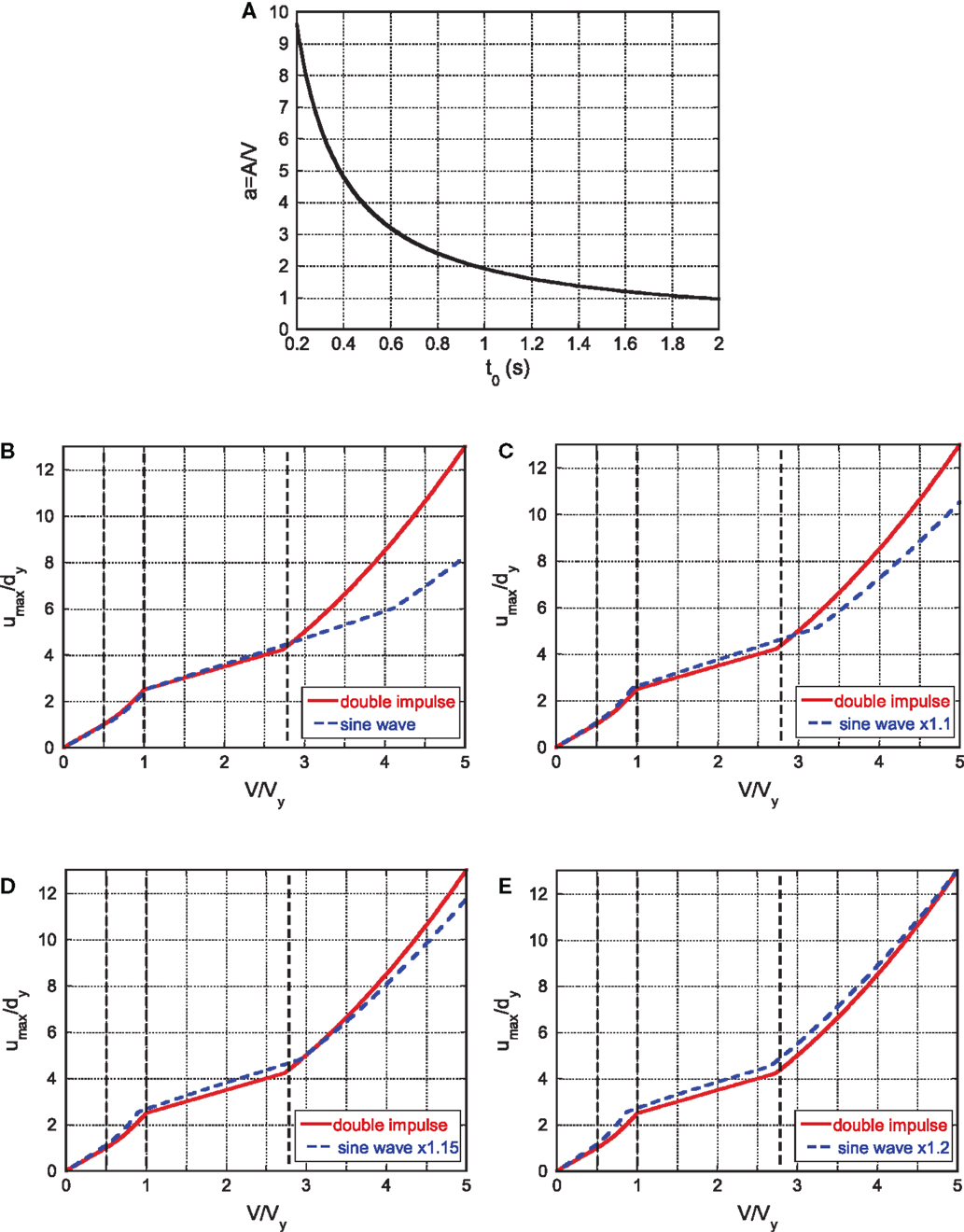Figure 13. Better correspondence of maximum responses to double impulse and amplified sinusoidal inputs: (A) plot of the coefficient a with respect to the timing of the double impulse for adjusting the maximum Fourier amplitudes of the double impulse and the sinusoidal input, (B) amplification factor = 1.0, (C) amplification factor = 1.1, (D) amplification factor = 1.15, and (E) amplification factor = 1.2.

## Applicability to Recorded Ground Motions

It seems important to investigate the applicability of the present theory to actual recorded pulse-type ground motions. As explained in the previous sections, the maximum deformation of the simplified SR model can be obtained from the equivalent SDOF model. Figure 7 can be used for estimating the maximum deformation of the equivalent SDOF model. Therefore, it is sufficient to investigate the response of the SDOF model. Moreover, since the consideration of ground conditions in actual recorded ground motions is complicated, an SDOF model with fixed base is considered in this section.

Consider two representative pulse-type ground motions, the Rinaldi station fault-normal component during the Northridge earthquake in 1994 and the Kobe University NS component (almost fault-normal) during the Hyogoken-Nanbu (Kobe) earthquake in 1995. Since the ground motions are fixed, the structural models are varied, i.e., ω1 or dy in Vy = ω1dy is varied. Figure 14 illustrates the modeling of the part of the recorded ground motion acceleration into a one-cycle sinusoidal input. Figure 15 shows the maximum amplitude of deformation for the recorded ground motions and the corresponding proposed one. As stated before, since the initial velocity V is determined in Figure 14, Vy is changed here. Because ω1 is closely related to the resonance condition, dy is changed principally. This procedure is similar to the well-known elastic–plastic response spectrum developed in 1960–1970. The solid line is obtained by changing Vy for the specified V using the method for the double impulse and the dotted line is drawn by conducting the elastic–plastic time-history response analysis on each model with varied Vy under the recorded ground motion. It can be observed that the result by the proposed method is a fairly good approximate of the recorded pulse-type ground motions.

FIGURE 14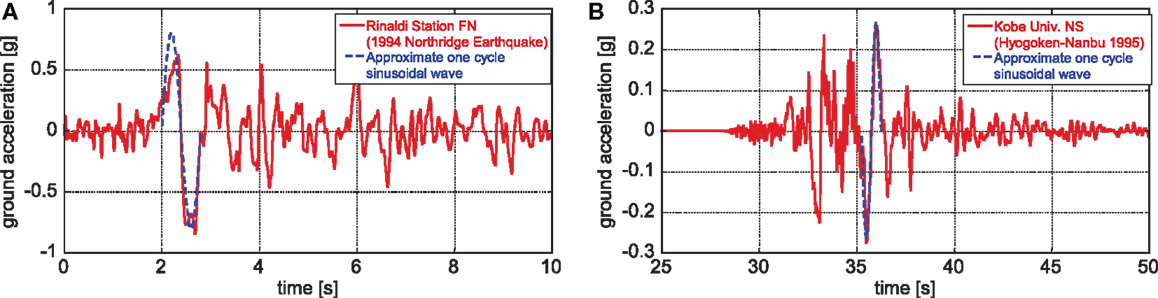Figure 14. Modeling of part of pulse-type recorded ground motion into the corresponding one-cycle sinusoidal input: (A) Rinaldi station fault-normal component during the Northridge earthquake in 1994 and (B) Kobe University NS component (almost fault-normal) during the Hyogoken-Nanbu (Kobe) earthquake in 1995.

FIGURE 15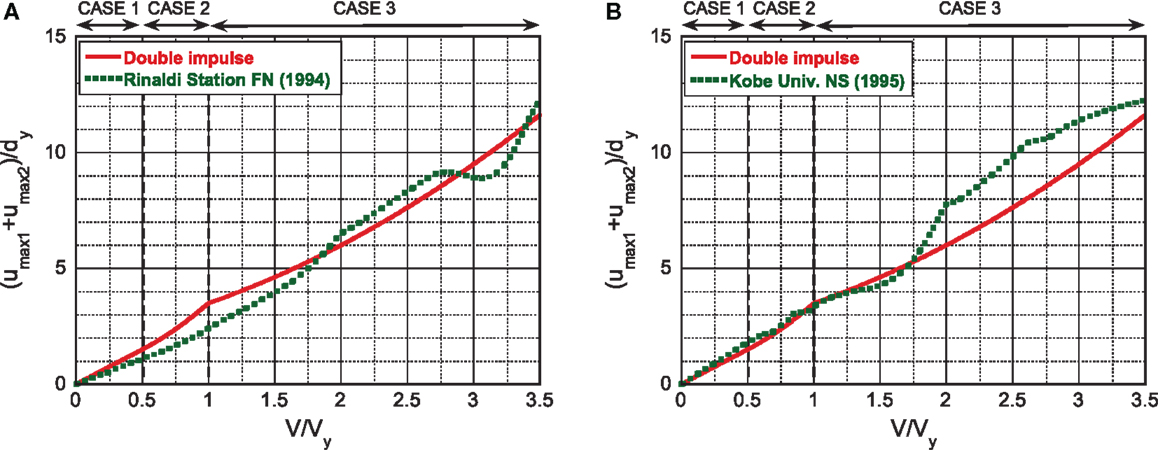Figure 15. Maximum amplitude of deformation for the recorded ground motions and the proposed one: (A) Rinaldi station fault-normal component and (B) Kobe University NS component.

## Conclusion

The double impulse has been introduced as a substitute of the fling-step near-fault ground motion. A closed-form solution of the elastic–plastic response of a structure on compliant (flexible) ground by the “critical double impulse” is derived for the first time based on the solution for the corresponding structure with fixed base. The detailed conclusions may be summarized as follows:

(1) The expression for a closed-form solution of the elastic–plastic response of an SDOF model by the critical double impulse has been extended to a simplified SR model. The simplified SR model can be derived by neglecting the foundation mass and floor mass moments of inertia in the original SR model.

(2) The simplified SR model has been transformed into an equivalent SDOF model with only one equivalent spring, which was modeled from three springs (superstructure, swaying, and rocking) via static condensation. By applying the previous work for an SDOF model to the equivalent SDOF model, the maximum elastic–plastic response of the equivalent SDOF model under the critical double impulse has been derived for the first time in closed form, and the corresponding elastic–plastic deformation of the superstructure has been evaluated. It is remarkable that no iteration is needed in the derivation of the critical elastic–plastic response.

(3) By taking full advantage of the closed-form expression of the critical elastic–plastic response of the superstructure, the relation of the critical elastic–plastic response of the superstructure with the ground stiffness has been clarified. It has been shown that in the case of a smaller input level of double impulse to the structural strength, as the ground stiffness becomes larger, the maximum plastic deformation also becomes larger. On the other hand, in the case of a larger input level of double impulse to the structural strength, as the ground stiffness becomes smaller, the maximum plastic deformation becomes larger. This property can be explained by taking into account the elastic strain energy stored in the swaying and rocking springs. In the smaller input level (Case 2), as the ground stiffness becomes larger, the ground deformation after the second impulse becomes smaller and the elastic strain energy stored in the swaying and rocking springs becomes smaller. Then the plastic deformation of the superstructure becomes larger. On the other hand, in the larger input level (Case 3), as the ground stiffness becomes smaller, the elastic strain energy stored in the swaying and rocking springs during the plastic deformation in the superstructure after the first impulse becomes larger. This elastic strain energy stored in the swaying and rocking springs during the plastic deformation in the superstructure plays an important role in the magnitude of plastic deformation of the superstructure after the second impulse.

(4) It has been demonstrated that the critical timing derived from the double impulse is also an approximate critical timing of the sinusoidal input.

(5) In the input range of V/Vy > 3, the maximum response of the double impulse becomes larger than that of the sinusoidal input. The better correspondence over a wider input range can be achieved by amplifying the amplitude of the sinusoidal input. The amplification factor 1.15 or 1.2 provides the best fitting.

(6) It has been demonstrated that the proposed method using the double impulse is applicable to actual recorded pulse-type ground motions within a reasonable accuracy.

The property in Conclusion (3) indicates that when we design a building on a soft ground, we have to design stronger members in such a building. On the contrary, it is generally believed that the soil–structure interaction can reduce the building response under earthquake ground motion. The period of pulse-like waves is in the range of 0.5–3 s. Since the critical input means the resonant case, the present theory dealing with the resonant response should be applied to buildings except very flexible ones of which the fundamental natural period is longer than 3 s.

## Author Contributions

KK developed the theory, conducted the analysis, and wrote the paper. IT supervised the research and wrote the paper.

## Conflict of Interest Statement

The authors declare that the research was conducted in the absence of any commercial or financial relationships that could be construed as a potential conflict of interest.

## Funding

Part of the present work is supported by the Grant-in-Aid for Scientific Research of Japan Society for the Promotion of Science (No. 15H04079) and the 2013-MEXT-Supported Program for the Strategic Research Foundation at Private Universities in Japan. These supports are greatly appreciated.

## References

Alavi, B., and Krawinkler, H. (2004). Behaviour of moment resisting frame structures subjected to near-fault ground motions. Earthquake Eng. Struct. Dyn. 33, 687–706. doi: 10.1002/eqe.369

Bertero, V. V., Mahin, S. A., and Herrera, R. A. (1978). Aseismic design implications of near-fault San Fernando earthquake records. Earthquake Eng. Struct. Dyn. 6, 31–42. doi:10.1002/eqe.4290060105

Bray, J. D., and Rodriguez-Marek, A. (2004). Characterization of forward-­directivity ground motions in the near-fault region. Soil Dyn. Earthquake Eng. 24, 815–828. doi:10.1016/j.soildyn.2004.05.001

Caughey, T. K. (1960a). Sinusoidal excitation of a system with bilinear hysteresis. J. Appl. Mech. 27, 640–643. doi:10.1115/1.3644077

Caughey, T. K. (1960b). Random excitation of a system with bilinear hysteresis. J. Appl. Mech. 27, 649–652. doi:10.1115/1.3644077

Drenick, R. F. (1970). Model-free design of aseismic structures. J. Eng. Mech. Div. ASCE 96, 483–493.

Hall, J. F., Heaton, T. H., Halling, M. W., and Wald, D. J. (1995). Near-source ground motion and its effects on flexible buildings. Earthquake Spectra 11, 569–605. doi:10.1193/1.1585828

Hayden, C. P., Bray, J. D., and Abrahamson, N. A. (2014). Selection of near-fault pulse motions. J. Geotech. Geoenviron. Eng. 140, 04014030. doi:10.1061/(ASCE)GT.1943-5606.0001129

Jarernprasert, S., Bazan, E., and Bielak, J. (2013). Seismic soil-structure interaction response of inelastic structures. Soil Dyn. Earthquake Eng. 47, 132–143. doi:10.1016/j.soildyn.2012.08.008

Kalkan, E., and Kunnath, S. K. (2006). Effects of fling step and forward directivity on seismic response of buildings. Earthquake Spectra 22, 367–390. doi:10.1193/1.2192560

Kalkan, E., and Kunnath, S. K. (2007). Effective cyclic energy as a measure of seismic demand. J. Earthquake Eng. 11, 725–751. doi:10.1080/13632460601033827

Khaloo, A. R., Khosravi, H., and Hamidi Jamnani, H. (2015). Nonlinear interstory drift contours for idealized forward directivity pulses using “modified fish-bone” models. Adv. Struct. Eng. 18, 603–627. doi:10.1260/1369-4332.18.5.603

Kojima, K., Fujita, K., and Takewaki, I. (2015). Critical double impulse input and bound of earthquake input energy to building structure. Front. Built Environ. 1:5. doi:10.3389/fbuil.2015.00005

Kojima, K., and Takewaki, I. (2015a). Critical earthquake response of elastic-plastic structures under near-fault ground motions (part 1: fling-step input). Front. Built Environ. 1:12. doi:10.3389/fbuil.2015.00012

Kojima, K., and Takewaki, I. (2015b). Critical earthquake response of elastic-plastic structures under near-fault ground motions (part 2: forward-directivity input). Front. Built Environ. 1:13. doi:10.3389/fbuil.2015.00012

Liu, C.-S. (2000). The steady loops of SDOF perfectly elastoplastic structures under sinusoidal loadings. J. Mar. Sci. Technol. 8, 50–60.

Makris, N., and Black, C. J. (2004). Dimensional analysis of rigid-plastic and elastoplastic structures under pulse-type excitations. J. Eng. Mech. ASCE 130, 1006–1018. doi:10.1061/(ASCE)0733-9399(2004)130:9(1006)

Mavroeidis, G. P., Dong, G., and Papageorgiou, A. S. (2004). Near-fault ground motions, and the response of elastic and inelastic single-degree-freedom (SDOF) systems. Earthquake Eng. Struct. Dyn. 33, 1023–1049. doi:10.1002/eqe.391

Mavroeidis, G. P., and Papageorgiou, A. S. (2003). A mathematical representation of near-fault ground motions. Bull. Seismol. Soc. Am. 93, 1099–1131. doi:10.1785/0120020100

Minami, H., and Hayashi, Y. (2013). Response characteristics evaluation of elastic shear beam for pulse waves. J. Struct. Constr. Eng. AIJ 685, 453–461. doi:10.3130/aijs.78.453

Moustafa, A., Ueno, K., and Takewaki, I. (2010). Critical earthquake loads for SDOF inelastic structures considering evolution of seismic waves. Earthquake Struct. 1, 147–162. doi:10.12989/eas.2010.1.2.147

Mukhopadhyay, S., and Gupta, V. K. (2013a). Directivity pulses in near-fault ground motions – I: identification, extraction and modeling. Soil Dyn. Earthquake Eng. 50, 1–15. doi:10.1016/j.soildyn.2013.02.017

Mukhopadhyay, S., and Gupta, V. K. (2013b). Directivity pulses in near-fault ground motions – II: estimation of pulse parameters. Soil Dyn. Earthquake Eng. 50, 38–52. doi:10.1016/j.soildyn.2013.02.019

Parmelee, R. A. (1970). “The influence of foundation parameters on the seismic response of interaction systems,” in Proceedings of the 3rd Japan Earthquake Engineering Symposium, Vol. 3 (Tokyo), 49–56.

Roberts, J. B., and Spanos, P. D. (1990). Random Vibration and Statistical Linearization. New York: Wiley.

Rupakhety, R., and Sigbjörnsson, R. (2011). Can simple pulses adequately represent near-fault ground motions? J. Earthquake Eng. 15, 1260–1272. doi:10.1080/13632469.2011.565863

Sasani, M., and Bertero, V. V. (2000). “Importance of severe pulse-type ground motions in performance-based engineering: historical and critical review,” in Proceedings of the Twelfth World Conference on Earthquake Engineering (Auckland).

Takewaki, I. (2002). Robust building stiffness design for variable critical excitations. J. Struct. Eng. ASCE 128, 1565–1574. doi:10.1061/(ASCE)0733-9445(2002)128:12(1565)

Takewaki, I. (2007). Critical Excitation Methods in Earthquake Engineering (Second Edition in 2013). Amsterdam: Elsevier.

Takewaki, I., Moustafa, A., and Fujita, K. (2012). Improving the Earthquake Resilience of Buildings: The Worst Case Approach. London: Springer.

Takewaki, I., and Tsujimoto, H. (2011). Scaling of design earthquake ground motions for tall buildings based on drift and input energy demands. Earthquake Struct. 2, 171–187. doi:10.12989/eas.2011.2.2.171

Vafaei, D., and Eskandari, R. (2015). Seismic response of mega buckling-­restrained braces subjected to fling-step and forward-directivity near-fault ground motions. Struct. Design Tall Spec. Build. 24, 672–686. doi:10.1002/tal.1205

Veletsos, A. S. (1977). “Dynamics of structure-foundation systems,” in Structural and Geotechnical Mechanics, A Volume Honoring N. M. Newmark, ed. Hall W. J. (Englewood Cliffs, NJ: Prentice-Hall), 333–361.

Veletsos, A. S., and Meek, J. W. (1974). Dynamic behaviour of building-­foundation systems. Earthquake Eng. Struct. Dyn. 3, 121–138. doi:10.1002/eqe.4290030203

Xu, Z., Agrawal, A. K., He, W.-L., and Tan, P. (2007). Performance of passive energy dissipation systems during near-field ground motion type pulses. Eng. Struct. 29, 224–236. doi:10.1016/j.engstruct.2006.04.020

Yamamoto, K., Fujita, K., and Takewaki, I. (2011). Instantaneous earthquake input energy and sensitivity in base-isolated building. Struct. Design Tall Spec. Build. 20, 631–648. doi:10.1002/tal.539

Yang, D., and Zhou, J. (2014). A stochastic model and synthesis for near-fault impulsive ground motions. Earthquake Eng. Struct. Dyn. 44, 243–264. doi:10.1002/eqe.2468

Zhai, C., Chang, Z., Li, S., Chen, Z.-Q., and Xie, L. (2013). Quantitative identification of near-fault pulse-like ground motions based on energy. Bull. Seism. Soc. Am. 103, 2591–2603. doi:10.1785/0120120320

Keywords: earthquake response, critical response, elastic–plastic response, soil–structure interaction, near-fault ground motion, fling-step input, double impulse

Citation: Kojima K and Takewaki I (2016) Closed-Form Critical Earthquake Response of Elastic–Plastic Structures on Compliant Ground under Near-Fault Ground Motions. Front. Built Environ. 2:1. doi: 10.3389/fbuil.2016.00001

Received: 24 November 2015; Accepted: 04 January 2016;
Published: 22 January 2016

Edited by:

Solomon Tesfamariam, The University of British Columbia, Canada

Reviewed by:

Hossein Mostafaei, FM Global, USA
Rola Assi, École de Technologie Supérieure, Canada

Copyright: © 2016 Kojima and Takewaki. This is an open-access article distributed under the terms of the Creative Commons Attribution License (CC BY). The use, distribution or reproduction in other forums is permitted, provided the original author(s) or licensor are credited and that the original publication in this journal is cited, in accordance with accepted academic practice. No use, distribution or reproduction is permitted which does not comply with these terms.

*Correspondence: Izuru Takewaki, takewaki@archi.kyoto-u.ac.jp